• 1.对前几部得到的特征进行分类，主要用到sklearn中的LightGBM进行评估，并用网格搜索进行参数调优。 2.Lightgbm是2017年在当时的NeurIPS（当时为NIPS）上发表的论文，文中主要是相比于XGBoost，LightGBM更高效。 ...

1.对前几部得到的特征进行分类，主要用到sklearn中的LightGBM进行评估，并用网格搜索进行参数调优。

2.Lightgbm是2017年在当时的NeurIPS（当时为NIPS）上发表的论文，文中主要是相比于XGBoost，LightGBM更高效。

import pandas as pd
from sklearn.model_selection import GridSearchCV
import lightgbm as lgb
from sklearn.metrics import f1_score
from sklearn.feature_extraction.text import TfidfVectorizer

"""读取数据"""
train_data.drop(columns=['article','id'], inplace = True)
test_data.drop(columns=['article'], inplace = True)

"""提取特征并划分数据集"""
tfidf=TfidfVectorizer()
x_train=tfidf.fit_transform(train_data['word_seg'])

x_train, x_test, y_train, y_test = train_test_split(x_train, Y_train, test_size=0.25, random_state=33)

"""训练和验证"""
lightgbm  = lgb.sklearn.LGBMClassifier()
param_grid = {
'learning_rate': [0.01, 0.1, 0.5],
'n_estimators': [30, 40]
}
lightgbm = GridSearchCV(lightgbm, param_grid)
lightgbm.fit(x_train, y_train)
y_lgb = lightgbm.predict(x_test)
print(f1_score(y_test, y_lgb , average='weighted'))

最后的结果为：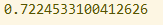展开全文• 中文文档：http://lightgbm.apachecn.org/cn/latest/Parameters.html #调用sklearn库中的指标求解 from sklearn import metrics from sklearn.metrics import precision_recall_curve from sklearn.metrics import...

#调用sklearn库中的指标求解
from sklearn import metrics
from sklearn.metrics import precision_recall_curve
from sklearn.metrics import average_precision_score
from sklearn.metrics import accuracy_score
print("accuracy_score:", accuracy_score(test_y, results))
print("precision_score:", metrics.precision_score(test_y, results))
print("recall_score:", metrics.recall_score(test_y, results))
print("f1_score:", metrics.f1_score(test_y, results))
print("f0.5_score:", metrics.fbeta_score(test_y, results, beta=0.5))
print("f2_score:", metrics.fbeta_score(test_y, results, beta=2.0))
展开全文• You can find the full ...具体可以看文章:Survival Analysis with LightGBM plus Poisson Regression https://towardsdatascience.com/survival-analysis-with-lightgbm-plus-poisson-regression-6b3cc897af82 1 Po

来看一个比较特殊的Survival分析建模的案例,利用的是半参模型:Poisson Regression
具体参考文章:Survival Analysis with LightGBM plus Poisson Regression
里面的建模思路非常有意思，不适合工业落地，不过咨询公司的data scientist看过来~

1 Poisson Regression

1.1 松泊分布与泊松回归

试想一下，你现在就站在一个人流密集的马路旁，打算收集闯红灯的人群情况（？）。
首先，利用秒表和计数器，一分钟过去了，有5个人闯红灯；
第二分钟有4个人；而下一分钟有4个人。
持续记录下去，你就可以得到一个模型，这便是“泊松分布”的原型。

除此以外，现实生活中还有很多情况是服从泊松分布的：

• 10分钟内从ATM中取钱的人数
• 一天中发生车祸的次数
• 每100万人中患癌症的人数
• 单位面积土地内昆虫的数目

Poisson模型(泊松回归模型)是用于描述单位时间、单位面积或者单位容积内某事件发现的频数分布情况，
通常用于描述稀有事件（即小概率）事件发生数的分布。

上述例子中都明显的一个特点：
低概率性，以及单位时间(或面积、体积)内的数量。
通常情况下，满足以下三个条件时，可认为数据满足Poisson分布：

• (1) 平稳性：发生频数的大小，只与单位大小有关系（比如1万为单位，或者100万为单位时患癌症人数不同）；
• (2) 独立性：发生频数的大小，各个数之间没有影响关系，即频数数值彼此独立没有关联关系；
比如前1小时闯红灯的人多了，第2小时闯红灯人数并不会受影响；
• (3) 普通性：发生频数足够小，即低概率性。

如果数据符合这类特征时，而又想研究X对于Y的影响（Y呈现出Poisson分布）；
此时则需要使用Poisson回归，而不是使用常规的线性回归等。

1.2 LightGBM 实现泊松回归的案例

参考来源：https://github.com/Microsoft/LightGBM/issues/807

import lightgbm as lgb
import numpy as np
import pandas as pd

n=100000
lam = .01
X = np.floor(np.random.lognormal(size=(n,2))).astype(int)
y = np.maximum(X[:,0],X[:,1])+np.random.poisson(lam=lam, size=n)

train_inds = np.arange(int(n/3))
val_inds =   np.arange(int(n/3), int(2*n/3))
test_inds =   np.arange(int(2*n/3), int(n))

X_test, y_test = X[test_inds,:], y[test_inds]

ds = lgb.Dataset(X,y, categorical_feature=)

ds_train = ds.subset(train_inds)
ds_val = ds.subset(val_inds)

params = {'objective':'poisson',
'metric':'rmse',
'learning_rate':.1
}
gbm = lgb.train(params, ds_train, num_boost_round=300, early_stopping_rounds=20, valid_sets=[ds_val, ds_train],
verbose_eval=100, categorical_feature=)

yhat = gbm.predict(X_test)
print('neg obs:', len(yhat[yhat<0]))

y是需要服从poisson分布的

2 数据解读

2.1 数据样式

数据集解释：美国Washington, D.C.的一个共享单车公司
数据来源：bike-sharing-dataset
数据集的中文字段解释：
参考：Capital Bikeshare （美国Washington, D.C.的一个共享单车公司）提供的自行车数据上进行回归分析
具体实现 + 代码：Survival_LGBM-github

# 字段说明Instant 记录号

Dteday：日期
Season：季节
1=春天
2=夏天
3=秋天
4=冬天
yr：年份，(0: 2011, 1:2012)
mnth：月份( 1 to 12)
hr：小时 (0 to 23) （只在 hour.csv 有，作业忽略此字段）
holiday：是否是节假日
weekday：星期中的哪天，取值为 0～6
workingday：是否工作日
1=工作日 （非周末和节假日）
0=周末
weathersit：天气
1：晴天，多云
2：雾天，阴天
3：小雪，小雨
4：大雨，大雪，大雾
temp：气温摄氏度
atemp：体感温度
hum：湿度
windspeed：风速

y值
-   casual：非注册用户个数
-  registered：注册用户个数
-  cnt：给定日期（天）时间（每小时）总租车人数，响应变量 y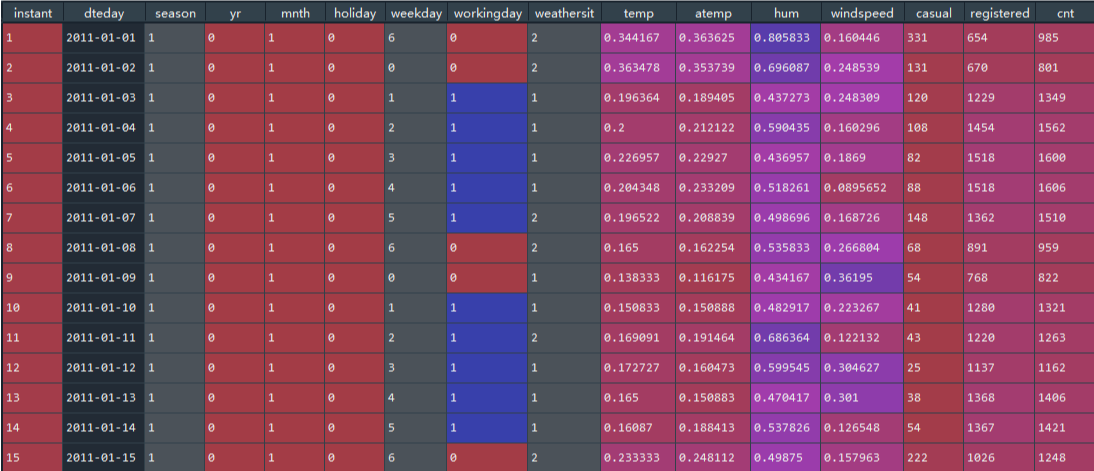现在数据变成：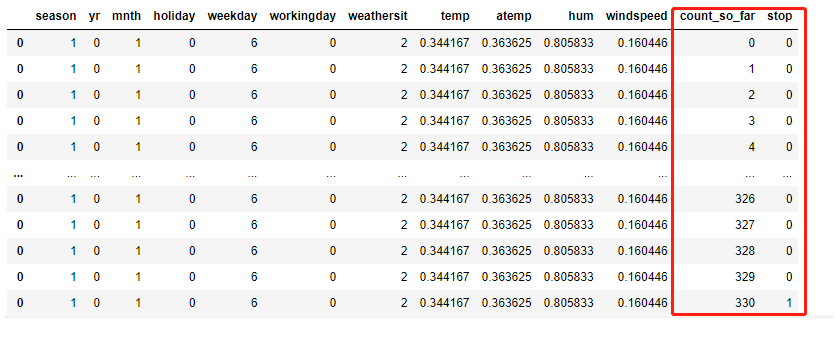也就是把第一行数据，拆分成330行，新增了两列：

• count_so_far就是人员计数从[0,1,2,3,4,6,…,330]，
• stop，就是人数终止计数

这样构造的一个特殊的数据结构，造就了该模型object的独特，原文自评:

We solve a not classical survival problem where we have not to estimate the probability of surviving past time, but we estimate the probability that a specific event occurs at the end of the day. This is a simple example but it shows how to applicate survival modeling techniques, with classical instruments, in a not common scenario where our duty is to estimate a probability density function.

这里就把问题变成了，2011/1/1 这天，
在casual users = 0的时候，不会停止增长；在casual users = 1，不会停止增长；…；在casual users = 330，停止增长
最后，如何来预测临时用户的人数？
用的是一个“可能在什么数量上停止”的曲线，这里是把 hazard = 临时用户的增长量，会拟合一个达到预测数值的可能性趋势。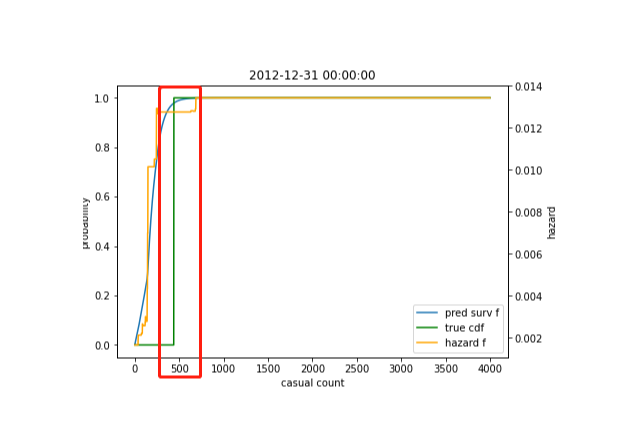这里跟生存分析 以及常规回归的差异：

• 常规回归模型，y~x1+x2… 根据特征直接预测临时用户人数，点估计；这里的Poisson有点类似加强版区间估计，就叫趋势估计？
• 生存分析，
• 生存函数使用的是KM曲线，计算不同生存时间区间下的存活率；
• 风险函数，计算不同生存时间区间下的风险值

2.2 衡量模型指标

2.2.1 CRPS

我们对结果的评价是用这种任务的标准分数来进行的。连续排序概率评分(Continuous ranking Probability Score, CRPS)将MAE推广到概率预测的情况。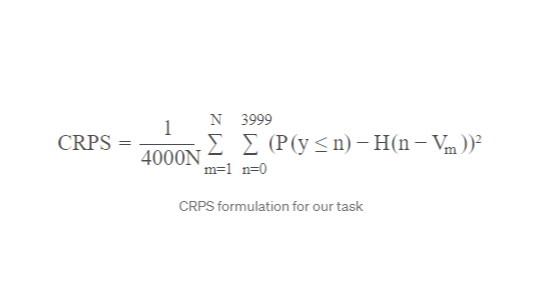在涉及概率预测的情况下，CPRS是应用最广泛的精度指标之一。它需要对所涉及的整个概率函数进行评估，因此需要对每个每日样本的整个生存曲线进行估计。

PDF / CDF

• 概率密度函数probability density function（pdf）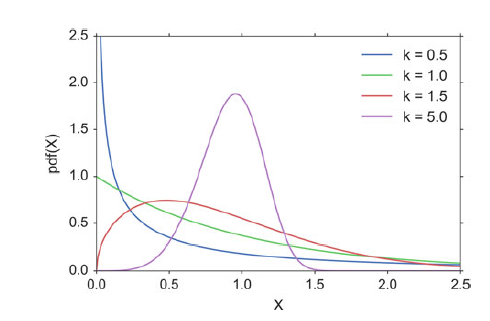• 累积分布函数(Cumulative Distribution Function)，又叫分布函数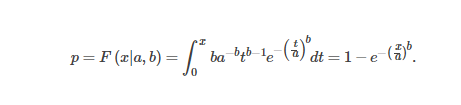2.2 训练与解读

数据的延展：

### EXPAND TRAIN DATA ###

X_train['count_so_far'] = X_train.apply(lambda x: np.arange(x.casual), axis=1)
X_train['stop'] = X_train.apply(lambda x: np.append(np.zeros(x.casual-1), 1), axis=1)
X_train = X_train.apply(pd.Series.explode)   # 数据扩充的方式

X_train['count_so_far'] = X_train.count_so_far.astype(int)
X_train['stop'] = X_train.stop.astype(int)

y_train = X_train['stop']
X_train = X_train[columns+['count_so_far']]

print(X_train.shape)
X_train

### EXPAND VALID DATA FOR PREDICTION ###

X_val_surv = df[(df.dteday >= datetime(year=2012, month=1, day=1))&(df.dteday < datetime(year=2012, month=5, day=1))].copy()

X_val_surv['count_so_far'] = X_val_surv.apply(lambda x: np.arange(max_count), axis=1)
X_val_surv['stop'] = X_val_surv.apply(lambda x:np.append(np.zeros(x.casual), np.ones(max_count-x.casual)), axis=1)
X_val_surv = X_val_surv.apply(pd.Series.explode)

X_val_surv['count_so_far'] = X_val_surv.count_so_far.astype(int)
X_val_surv['stop'] = X_val_surv.stop.astype(int)

y_val_surv = X_val_surv['stop']
X_val_surv = X_val_surv[columns+['count_so_far']]

print(X_val_surv.shape)

具体的已经在2.1 描述过了；这里要额外来看一下max_count，这个在train数据集中不会出现，
也就是今天有多少临时用户，就是多少X_train.apply(lambda x: np.arange(x.casual), axis=1)
但你会看到，X_val_surv是X_val_surv.apply(lambda x: np.arange(max_count), axis=1)，这里就是临时用户的最大值设定为4000，这个值根据临时用户max值来取的，就是临时用户上线。

params = {
'objective':'poisson',
'num_leaves':30,
'learning_rate': 0.001,
'feature_fraction': 0.8,
'bagging_fraction': 0.9,
'bagging_seed': 33,
'poisson_max_delta_step': 0.8,
'metric': 'poisson'
}

### FIT LGBM WITH POISSON LOSS ###

trn_data = lgb.Dataset(X_train, label=y_train)
val_data = lgb.Dataset(X_val, label=y_val)

model = lgb.train(params, trn_data, num_boost_round=1000,
valid_sets = [trn_data, val_data],
verbose_eval=50, early_stopping_rounds=150)

这里X_train的shape为：(247252, 13)，那么可以知道训练集是非常大的；
需要重置数据，可到：

### PREDICT HAZARD FUNCTION ON VALIDATION DATA AND TRANSFORM TO SURVIVAL ###

p_val_hz = model.predict(X_val_surv).reshape(-1,max_count)
p_val = 1-np.exp(-np.cumsum(p_val_hz, axis=1))
X_val_surv.shape
>>> (484000, 12)
p_val.shape
>>> (121, 4000)

p_val就回归正常，代表着每一天，不同人群数量的概率，直接上图：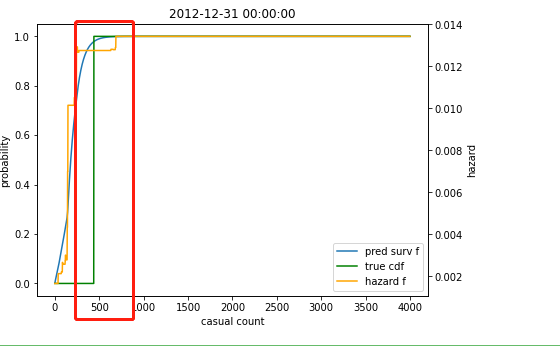这天在500左右，达到峰值了，那么这天临时用户的预测值就在500左右了。

简单的模型检验：

### CRPS ON VALIDATION DATA ###
crps(t_val, p_val)
>>> 0.17425293919055515
### CRPS ON VALIDATION DATA WITH BASELINE MODEL ###
crps(t_val, np.repeat(cdf, len(t_val)).reshape(-1,max_count))

3 同等lightGBM分类测试

https://blog.csdn.net/wang263334857/article/details/81836578

来看一下同一份数据测试出来的结果如何，后续不贴太多，只贴一下我测试的代码，放在了之前的一个项目下面：Survival_Poisson_Regression

展开全文泊松回归 生存分析 lightgbm 机器学习
• 文章目录lightgbm use_and_tuningusedata interface设置参数训练、预测tuning**ref：****note：**针对 Leaf-wise (最佳优先) 树的参数优化针对更快的训练速度针对更好的准确率处理过拟合 lightgbm use_and_tuning ...

use

两种的底层都是一致的

import lightgbm as lgb

import lightgbm as lgb

zh_doc python包的相关介绍

data interface

LightGBM Python 模块能够使用以下几种方式来加载数据:

• libsvm/tsv/csv txt format file（libsvm/tsv/csv 文本文件格式）
• Numpy 2D array, pandas object（Numpy 2维数组, pandas 对象）
• LightGBM binary file（LightGBM 二进制文件）

np 2D array形式：

data = np.random.rand(500, 10)  # 500 个样本, 每一个包含 10 个特征
label = np.random.randint(2, size=500)  # 二元目标变量,  0 和 1
train_data = lgb.Dataset(data, label=label)

还可以：指定 feature names（特征名称）和 categorical features（分类特征）、设置权重、分数等

【具体见文档zh_doc python包的相关介绍

设置参数

LightGBM 可以使用一个 pairs 的 list 或一个字典来设置参数. 例如:

• Booster（提升器）参数:

param = {'num_leaves':31, 'num_trees':100, 'objective':'binary'}
param['metric'] = 'auc'

• 您还可以指定多个 eval 指标:

param['metric'] = ['auc', 'binary_logloss']

训练、预测

训练

param_list、train_dataset是必需的

num_round = 10
bst = lgb.train(param, train_data, num_round, valid_sets=[test_data])

LightGBM 也支持加权训练，它需要一个额外的 加权数据 。 它需要额外的 query 数据 用于排名任务。

支持交叉验证

num_round = 10
lgb.cv(param, train_data, num_round, nfold=5)

支持提前停止

valid_sets：至少有一个. 如果有多个，它们都会被使用（如果有一个验证集）。

该模型将开始训练, 直到验证得分停止提高为止. 验证错误需要至少每个 early_stopping_rounds 减少以继续训练.

如果提前停止, 模型将有 1 个额外的字段: bst.best_iteration. 请注意 train() 将从最后一次迭代中返回一个模型, 而不是最好的一个.

如果您指定多个评估指标, 则它们都会用于提前停止.

bst = lgb.train(param, train_data, num_round, valid_sets=valid_sets, early_stopping_rounds=10)
bst.save_model('model.txt', num_iteration=bst.best_iteration)

预测

# 7 个样本, 每一个包含 10 个特征
data = np.random.rand(7, 10)
ypred = bst.predict(data)

如果在训练过程中启用了提前停止, 可以用 bst.best_iteration 从最佳迭代中获得预测结果:

ypred = bst.predict(data, num_iteration=bst.best_iteration)

模型保存

在训练完成后, 可以使用如下方式来存储模型:

bst.save_model('model.txt')

训练后的模型也可以转存为 JSON 的格式:

json_model = bst.dump_model()

以保存模型也可以使用如下的方式来加载.

bst = lgb.Booster(model_file='model.txt')  #init model

[具体见文档zh_doc python包的相关介绍]

同sklearn方式

fit函数包含了早停等

tuning

LightGBM 使用 leaf-wise 的树生长策略, 而很多其他流行的算法采用 depth-wise 的树生长策略. 与 depth-wise 的树生长策略相较, leaf-wise 算法可以收敛的更快. 但是, 如果参数选择不当的话, leaf-wise 算法有可能导致过拟合.

zh_doc 参数优化

note：

针对 Leaf-wise (最佳优先) 树的参数优化

1. num_leaves

控制树模型复杂度的主要参数。应让其小于2^(max_depth)，因为depth 的概念在 leaf-wise 树中并没有多大作用，并不存在从leavesdepth的映射

2. min_data_in_leaf

用于处理过拟合，该值取决于训练样本数和num_leaves，几百或几千即可。设置较大避免生成一个过深的树，可能导致欠拟合。

min_data_in_leaf, default=20, type=int, alias=min_data_per_leaf , min_data, min_child_samples

• 一个叶子上数据的最小数量. 可以用来处理过拟合.
3. max_depth

显示限制树的深度

针对更快的训练速度

• 通过设置 bagging_fractionbagging_freq 参数来使用 bagging 方法
• 通过设置 feature_fraction 参数来使用特征的子抽样
• 使用较小的 max_bin
• 使用 save_binary 在未来的学习过程对数据加载进行加速
• 使用并行学习, 可参考 并行学习指南

针对更好的准确率

• 使用较大的 max_bin （学习速度可能变慢）
• 使用较小的 learning_rate 和较大的 num_iterations
• 使用较大的 num_leaves （可能导致过拟合）
• 使用更大的训练数据
• 尝试 dart

处理过拟合

• 使用较小的 max_bin
• 使用较小的 num_leaves
• 使用 min_data_in_leafmin_sum_hessian_in_leaf
• 通过设置 bagging_fractionbagging_freq 来使用 bagging
• 通过设置 feature_fraction 来使用特征子抽样
• 使用更大的训练数据
• 使用 lambda_l1, lambda_l2min_gain_to_split 来使用正则
• 尝试 max_depth 来避免生成过深的树

具体调参方式总结

LightGBM 调参方法（具体操作）

LightGBM 训练及调参

zh_doc 参数

可以查看参数的别名和说明，如果调参出现重名，就会报warning。如：lambda_l1 is set=0, reg_alpha=0.0 will be ignored. Current value: lambda_l1=0

对于基于决策树的模型，调参的方法都是大同小异。一般都需要如下步骤：

1. 首先选择较高的学习率，大概0.1附近，这样是为了加快收敛的速度。这对于调参是很有必要的。
2. 对决策树基本参数调参
3. 正则化参数调参
4. 最后降低学习率，这里是为了最后提高准确率
other_params = {'num_leaves': 31, 'objective': None, 'learning_rate': 0.1, 'max_depth': -1, 'reg_alpha': 0.0,
'reg_lambda': 0.0, 'n_estimators': 100, 'boosting_type': 'gbdt', 'device': 'gpu',
'min_child_samples': 20, 'subsample': 1.0, 'subsample_freq': 0, 'colsample_bytree': 1.0,

'class_weight': None, 'importance_type': 'split',
'min_child_weight': 0.001, 'min_split_gain': 0.0, 'n_jobs': -1, 'random_state': None,
'subsample_for_bin': 200000, 'silent': True}

data_list_dict = get_data_np_dict(cell_name, feature_name, method_name)

# 第一次：max_depth、num_leaves
print("第一次")
cv_params = {'max_depth': [-1, 0, 3, 4, 5, 6, 7, 8], 'num_leaves': range(21, 200, 10)}
# cv_params = {'max_depth': range(3, 8, 4), 'num_leaves': range(5, 100, 45)}
best_params = lgb_grid_greedy(cv_params, other_params, '1')
other_params.update(best_params)

# 第二次
print("第二次")
cv_params = {'max_bin': range(5, 256, 10), 'min_child_samples': range(10, 201, 10)}
# cv_params = {'max_bin': range(5, 256, 100), 'min_child_samples': range(1, 102, 50)}
# cv_params = {'max_depth': [3, 4, ], 'min_child_weight': [1, 2, ]}
best_params = lgb_grid_greedy(cv_params, other_params, '2')
other_params.update(best_params)
# print(other_params)

# 第三次
print("第三次")
cv_params = {'colsample_bytree': [0.6, 0.7, 0.8, 0.9, 1.0],
'subsample': [0.6, 0.7, 0.8, 0.9, 1.0],
'subsample_freq': range(0, 81, 10)
}
# cv_params = {'colsample_bytree': [0.6, 0.7, ],
#              'subsample': [0.6, 0.7, ],
#              'subsample_freq': range(0, 81, 40)
#              }
best_params = lgb_grid_greedy(cv_params, other_params, '3')
other_params.update(best_params)
# print(other_params)

# 第四次
print("第四次")
cv_params = {'reg_alpha': [1e-5, 1e-3, 1e-1, 0.0, 0.1, 0.3, 0.5, 0.7, 0.9, 1.0],
'reg_lambda': [1e-5, 1e-3, 1e-1, 0.0, 0.1, 0.3, 0.5, 0.7, 0.9, 1.0]
}
# cv_params = {'reg_alpha': [1e-5, 1e-3, ],
#              'reg_lambda': [1e-5, 1e-3, ]
#              }
best_params = lgb_grid_greedy(cv_params, other_params, '4')
other_params.update(best_params)
# print(other_params)

# 第五次
print("第五次")
cv_params = {'min_split_gain': [0.0, 0.1, 0.2, 0.3, 0.4, 0.5, 0.6, 0.7, 0.8, 0.9, 1.0]}
# cv_params = {'min_split_gain': [0.0, 0.1, 0.2, 0.3, 0.4, 0.5, 0.6, 0.7, 0.8, 0.9, 1.0]}
best_params = lgb_grid_greedy(cv_params, other_params, '5')
other_params.update(best_params)
# print(other_params)

# 第六次
print("第六次")
cv_params = {'learning_rate': [0.001, 0.01, 0.05, 0.07, 0.1, 0.2, 0.5, 0.75, 1.0]}
# cv_params = {'learning_rate': [0.01, 0.05, ]}
best_params = lgb_grid_greedy(cv_params, other_params, '6')
other_params.update(best_params)
# print(other_params)

展开全文神经网络 机器学习 深度学习 lightgbmpyspark lightgbm mmlspark
• 二、数据读取 Baseline文档可以粗略的划分以下几部分： 工具包准备 import os import gc import math import pandas as pd import numpy as np import lightgbm as lgb # import xgboost as xgb ...数据挖掘 算法 python 机器学习 深度学习
• 文章目录一、LightGBM 原生接口重要参数训练参数预测方法绘制特征重要性分类例子回归例子二、LightGBM 的 sklearn 风格接口LGBMClassifier基本使用例子LGBMRegressor基本使用例子三、LightGBM 调参思路、参数网格...机器学习 python 算法
• LightGBM调参

千次阅读 2019-11-24 16:48:30
GBDT模型的另一进化版本：LightGBM。LigthGBM是boosting集合模型中的新进成员，由微软提供，它和XGBoost一样是对GBDT的高效实现，原理上它和GBDT及XGBoost类似，都采用损失函数的负梯度作为当前决策树的残差近似值...机器学习
• 评估指标 参赛队伍需要提交指定公司的二季度营收数据，以整数表示，精确到万元。该结果将与真实财报发布的数值进行对比。计算各个公司的相对预测误差，并进行对数市值加权，计算公式如下： 【赛题解析】 请参考： ...
• 基于以上三算法，LightGBM生产一片叶子需要的复杂度大大降低了，从而极大节约了计算时间。同时Histogram算法还将特征浮点数转换成0~255位的证书进行存储，从而集打节约了内存存储空间。 代码举例： impor机器学习 python
• 向AI转型的程序员都关注了这号????????????机器学习AI算法工程 公众号：datayxLearning to Rank 简介 去年实习时，因为项目需要，接触了一下Le...
• 超参数的调优（lightgbm)

千次阅读 2019-11-25 21:55:29
贝叶斯优化问题有四个组成部分： 目标函数：我们想要最小化的对象，这里指带超参数的机器学习模型的验证误差 域空间：待搜索的超参数值 优化算法：构造代理模型和选择接下来要评估的超参数值的方法 结果的.....调参
• XGBoost、LightGBM、Catboost总结

千次阅读 2019-03-02 20:25:40
是针对叶节点优先挑选不纯度下降最多的叶节点，这里有点LightGBM的’leaf-wise’的意味，而按树深分裂则更类似于原始的以及XGBoost的分裂方式) 学习率 learning_rate 对应取值范围在(0,1]之间的超参数对应GBRT...
• LightGBM调参指导 针对leaf-wise树的参数优化： num_leaves：控制了叶节点的数目。它是控制树模型复杂度的主要参数。 如果是level-wise，则该参数为2depth2depth，其中depth为树的深度。但是当叶子数量相同时，...数据挖掘 机器学习
• 白话机器学习算法理论+实战番外篇之LightGBM

千次阅读 多人点赞 2020-04-10 10:37:27
1. 写在前面 如果想从事数据挖掘或者机器学习的工作，掌握常用的机器学习算法是非常有必要的，在这简单...我前面已经尝试用最白话的语言完成了一白话机器学习算法理论+实战系列，这系列已经基本包含了上面这些算...机器学习算法 lightgbm xgboost
• 如果两个特征并不是完全互斥（部分情况下两个特征都是非零值），可以用一个指标对特征不互斥程度进行衡量，称之为冲突比率，当这个值较小时，我们可以选择把不完全互斥的两个特征捆绑，而不影响最后的精度。...算法 决策树 python 机器学习 人工智能
• 作者：黄雨龙，中国科学技术大学对于回归问题，Datawhale已经梳理过完整的实践方案（可点击），本文对多分类的数据挖掘问题做了完整的方案总结。一、赛题数据赛题背景本赛题是一多分类的数...数据挖掘 人工智能 python 机器学习 数据分析
• 目录 一、XGBoost参数解释 1.通用参数 ...LightGBM调参示例 五、XGBoost和LightGBM调参核心 调参方向：处理过拟合（过拟合和准确率往往相反） 调参范围 六、RandomForest参数解释 1....
• GBDT GBDT，也叫梯度提升决策树，英文叫Gradient Boosting Decision Tree，使用的是Boosting。...在GBDT中，每一分类器都会去拟合预测值与实际结果的残差，也就是误差，然后一层层地作用下来，最后根据每分...python 机器学习 人工智能 算法
• 基础模型建立2.1 LightGBM建模2.2 默认参数的效果三. 设置参数空间3.* 参数空间采样. 随机优化4.1 交叉验证LightGBM4.2 Objective Function4.3 执行随机调参4.4 Random Search 结果五. 贝叶斯优化5.1 Objective ...python 机器学习 贝叶斯优化 GBDT hyperopt
•  嗯嗯， 这其实就是下面要讲的lightgbm里面的直方图的思路， 所以直方图这思路在xgboost里面也体现过，不算是lightgbm的亮点了， 这是会有一些效果，可以减少点计算，但是比较微妙，lightgbm直方图算法进行了...
• Datawhale集成学习-XGBoost算法与LightGBM算法1.XGBoost算法2. Xgboost算法案例2.1 ## 分类案例2.1 回归案例2.3 XGBoost调参（结合sklearn网格搜索）3. LightGBM算法 1.XGBoost算法   XGBoost是陈天奇等人开发的一...算法 机器学习 python
• 【机器学习】基于逻辑回归，LightGBM,XGBoost额的分类预测一.基于逻辑回归的分类预测1 逻辑回归的介绍和应用1.1 逻辑回归的介绍1.2逻辑回归的应用2.Demo实践**Step1:库函数导入****Step2:模型训练****Step3:模型参数...逻辑回归 分类 机器学习
• 许多利用GBDT技术的算法（例如，XGBoost、LightGBM），构建下一棵树分为两阶段：选择树结构和在树结构固定后计算叶子节点的值。为了选择最佳的树结构，算法通过枚举不同的分割，用这些分割构建树，对得到的叶子...算法 大数据 机器学习 人工智能 python
• LightGBM 调参

2020-12-08 18:00:40
文章目录一、LightGBM简介二、LightGBM原理2.1 直方图算法2.2 LightGBM的直方图做差加速2.3 带深度限制的Leaf-wise的叶子生长策略3.4 直接支持类别特征（即不需要做one-hot编码）3.5 直接支持高效并行三、梯度提升的...
• 1.概述 RF、GBDT和都属于集成学习... xgboost和lightgbm是gbdt的优秀工程实现及优化改进。 2.集成学习方法 bootstrap, boosting, bagging, stacking集成算法原理介绍 详细的bootstrap 3.各种实例解析 ...
• 介绍了如何通过DaaS-Client Python客户端自动部署开源AI模型到生产环境中：Sklearn、XGBoost、LightGBM和PySpark，并且在DaaS网络客户端管理这些模型和部署AI 模型部署...